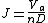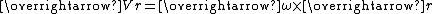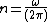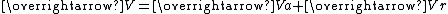xAdvance ratioEncyclopedia
In aeronautics
Aeronautics
Aeronautics is the science involved with the study, design, and manufacturing of airflight-capable machines, or the techniques of operating aircraft and rocketry within the atmosphere...

and marine hydrodynamics, the advance ratio at which a propeller
Propeller
A propeller is a type of fan that transmits power by converting rotational motion into thrust. A pressure difference is produced between the forward and rear surfaces of the airfoil-shaped blade, and a fluid is accelerated behind the blade. Propeller dynamics can be modeled by both Bernoulli's...

is operating is the ratio between the distance the propeller moves forward through the fluid during one revolution, and the diameter of the propeller. When a propeller-driven vehicle is moving at high speed relative to the fluid the advance ratio of its propeller(s) is a high number; and when it is moving at low speed the advance ratio is a low number. The advance ratio of a propeller is analogous to the angle of attack
Angle of attack
Angle of attack is a term used in fluid dynamics to describe the angle between a reference line on a lifting body and the vector representing the relative motion between the lifting body and the fluid through which it is moving...

of an airfoil
Airfoil
An airfoil or aerofoil is the shape of a wing or blade or sail as seen in cross-section....

.

## Advance ratio

The advance ratio J is given by:where
 Va is the speed of advance, typically the true airspeedTrue airspeedTrue airspeed of an aircraft is the speed of the aircraft relative to the airmass in which it is flying. True airspeed is important information for accurate navigation of an aircraft.-Performance:... of the aircraft or the water speed of the vessel n is the propeller's rotational speed in revolutions per unit of time D is the propeller's diameter

The advance ratio is a means of describing the incoming angle of the fluid relative to the propeller blade.

## Fluid velocity

The fluid velocity relative to the propeller blade increases with distance from the hub, due to the rotational velocity of the propeller. Therefore, the direction of the flow relative to the propeller blade is equal to the vector sum of the speed of advance and the rotational speed of the propeller. In mathematical terms,where
 Vr is the linear velocity vector of the propeller blade at distance r from its center. ω is the propeller's rotational speed in radians per unit of time V is the vector of the incoming fluid flow relative to the propeller blade at distance r from its center r is the distance from the center of the propeller to the radial point of evaluation, 0<=r<=R R is the radius of the propeller

Any propeller, be it for marine or aeronatical use, has blades shaped like airfoils, which twist towards the direction of rotation with increasing distance from the hub. This is to produce a relatively constant "angle of attack" for the blades at the speed of advance for which that particular propeller was designed.
It is worth noting that the formula for the advance ratio is remarkably similar to the ratio of Va/Vr, the difference being a constant of value r/(D*2π), considering that Va and Vr are orthogonal by design, Va/Vr is the tangent of the incoming angle of the fluid relative to the propeller blade. It is for that reason that the advance ratio is in fact analogous to angle of attack for propeller blades.
It is also important to note that the advance ratio J is a non-dimensional parameter, and as such all units on the right side of the equation should cancel out. If the speed of advance is measured in feet per second, for example, the rotational speed must be measured in revolutions per second and the diameter must be measured in feet. The performance of different propellers should be compared for the same advance ratio.

## External links

The source of this article is wikipedia, the free encyclopedia.  The text of this article is licensed under the GFDL.